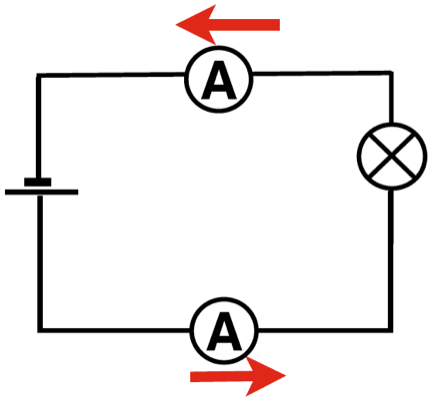Kirchoff’s First Law in a series circuit

In a series circuit the same current flows all the way around. The position of the ammeter is unimportant because it will read the same wherever it is placed.In a branched circuit

The most often used and complete statement of Kirchoff’s First Law states is that vector sum of the current at any point in a circuit is zero.

Another way of saying this (but not the proper statement is that:

In a series circuit the same current flows all the way around. It does not matter where it is measured it will be the same at every point.

In a branched or parallel circuit the current will split between the branches so that if we add up the current measurement in each branch it will be the same as in the main part of the circuit.

If we use the proper statement to analyse the current in the branched (parallel) circuits below. If the current going into point W is positive and that leaving is negative, then from the circuit here:

0.48 - 0.25 - 0.23 = 0

Watch the video to see these measurements in a more practical way.